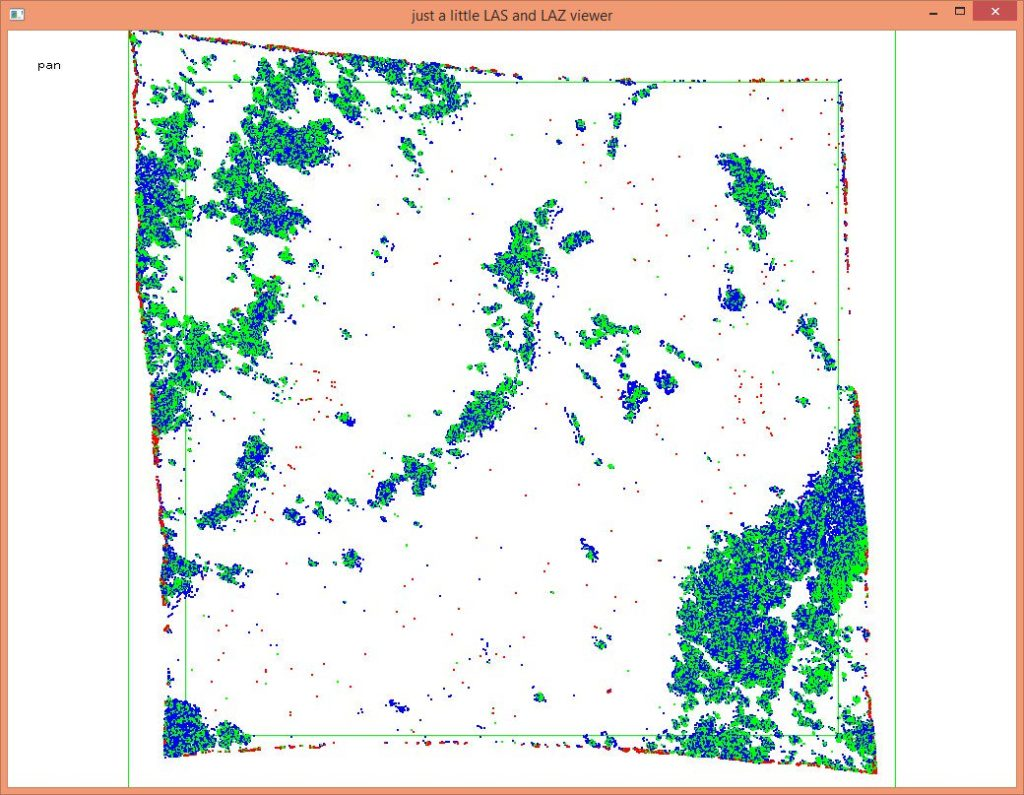# lasreturn

reads LAS or LAZ files containing points from multi-return LiDAR systems and either reports return statistics, find and marks sets of points from the same laser shots that are incomplete (i.e. missing returns), or repairs the ‘number of returns’ field based on GPS times. Note that input files need to be sorted based on their GPS time stamp, which can be done with lassort. For more details see the README file. Below an example output from a user forum post that indicates trouble:

``````lasreturn -i tile_sorted.laz ^
-check_return_numbering
checked returns of 341230 multi and 824 single return pulses. took 0.511 secs
missing: 601 duplicate: 431281 too large: 0 zero: 0
missing
=======
25 returns with n = 2 and r = 1 are missing
520 returns with n = 2 and r = 2 are missing
6 returns with n = 3 and r = 1 are missing
24 returns with n = 3 and r = 2 are missing
26 returns with n = 3 and r = 3 are missing
duplicate
========
253961 returns with n = 1 and r = 1 are duplicate
83775 returns with n = 2 and r = 1 are duplicate
83278 returns with n = 2 and r = 2 are duplicate
3404 returns with n = 3 and r = 1 are duplicate
3389 returns with n = 3 and r = 2 are duplicate
3386 returns with n = 3 and r = 3 are duplicate
22 returns with n = 4 and r = 1 are duplicate
22 returns with n = 4 and r = 2 are duplicate
22 returns with n = 4 and r = 3 are duplicate
22 returns with n = 4 and r = 4 are duplicate``````

In another example we found many cut-off tree tops in some LiDAR tile that we then visualized with lasview by marking the sets of points that were not complete (i.e. had missing returns) with a special classification code.

``````lasreturn -i tile_sorted.laz ^
-check_return_numbering ^
-classify_as 8 ^
-odix _marked -olaz

lasview -i tile_sorted_marked.laz ^
-keep_class 8``````These points are incomplete sets of returns, often hinting at a processing error (unless at the edge of a tile).
Nach oben scrollen